# 3 6 Ratios and Proportions Objective Students will

• Slides: 13
Download presentation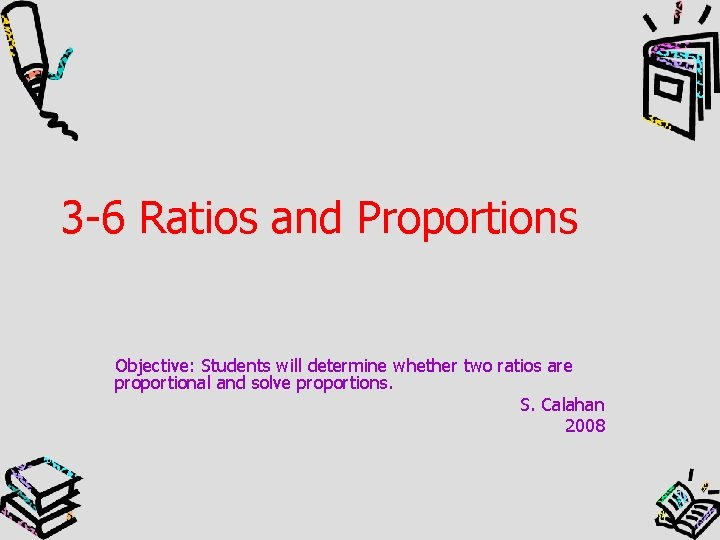3 -6 Ratios and Proportions Objective: Students will determine whether two ratios are proportional and solve proportions. S. Calahan 2008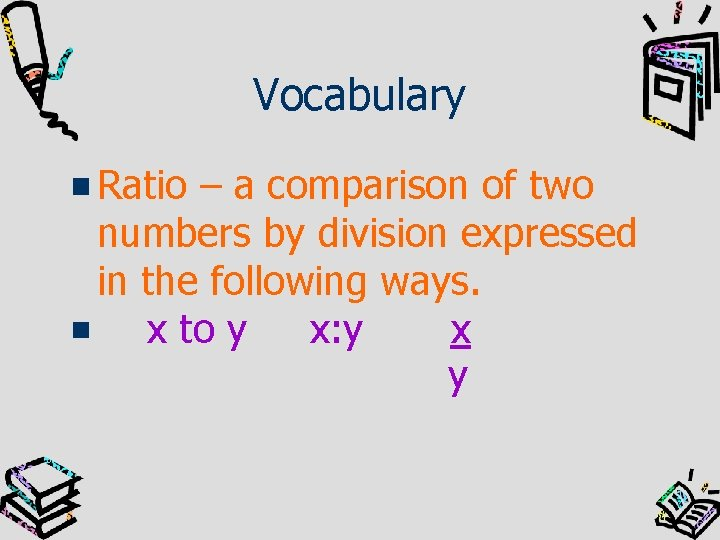Vocabulary Ratio – a comparison of two numbers by division expressed in the following ways. x to y x: y x yProportion An equation stating that two ratios are equal. 2=4 6 12Determine whether ratios form a proportion 4 and 24 5 30 determine if the larger ratio can be reduced to equal the smaller ratio 24 ÷ 6 = 4 30 ÷ 6 = 5 Yes, the ratios are equal, therefore they form a proportion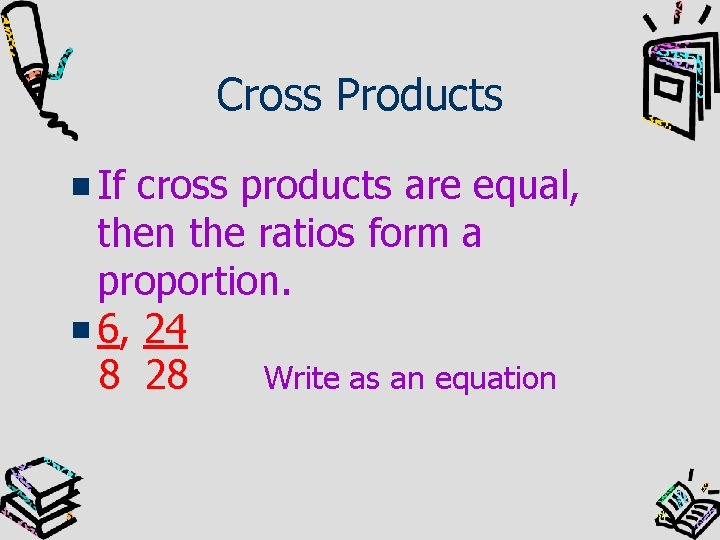Cross Products If cross products are equal, then the ratios form a proportion. 6, 24 8 28 Write as an equation6 = 24 8 28 Find the cross products. 6(28) = 8(24) 168 = 192 Since the cross products are not equal, the ratios are not proportional.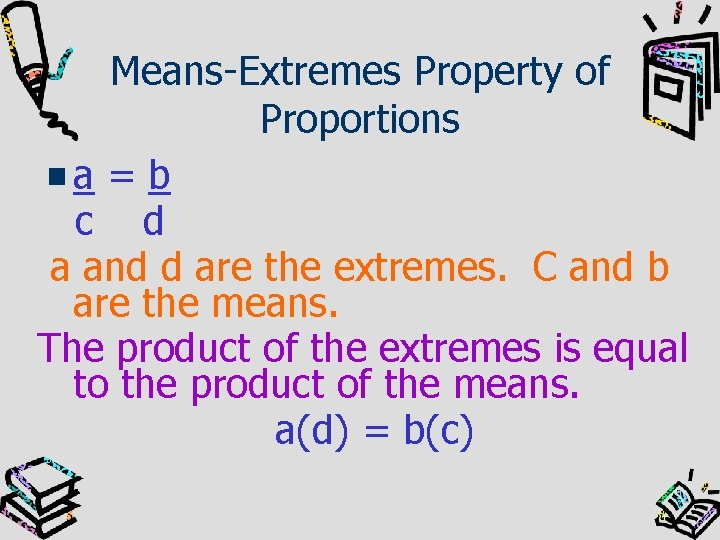Means-Extremes Property of Proportions a=b c d a and d are the extremes. C and b are the means. The product of the extremes is equal to the product of the means. a(d) = b(c)Solve a proportion n = 24 15 16 16(n) = 15(24) 16 n = 360 16 16 n = 22. 5 find cross products divide both sides by 16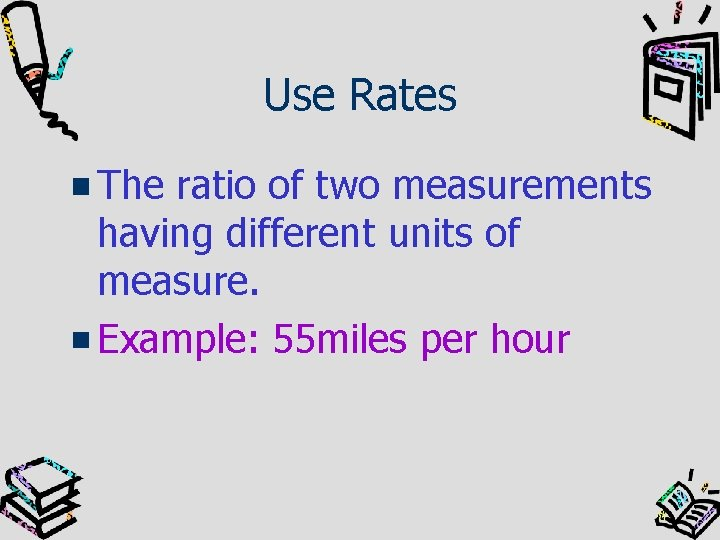Use Rates The ratio of two measurements having different units of measure. Example: 55 miles per hour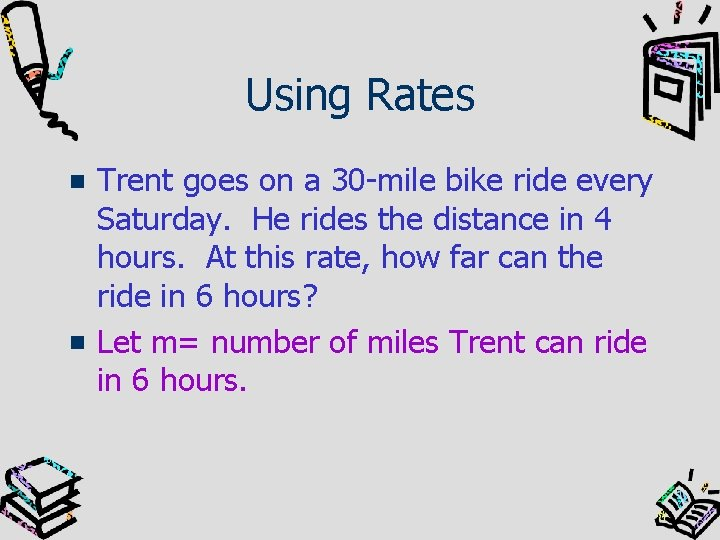Using Rates Trent goes on a 30 -mile bike ride every Saturday. He rides the distance in 4 hours. At this rate, how far can the ride in 6 hours? Let m= number of miles Trent can ride in 6 hours.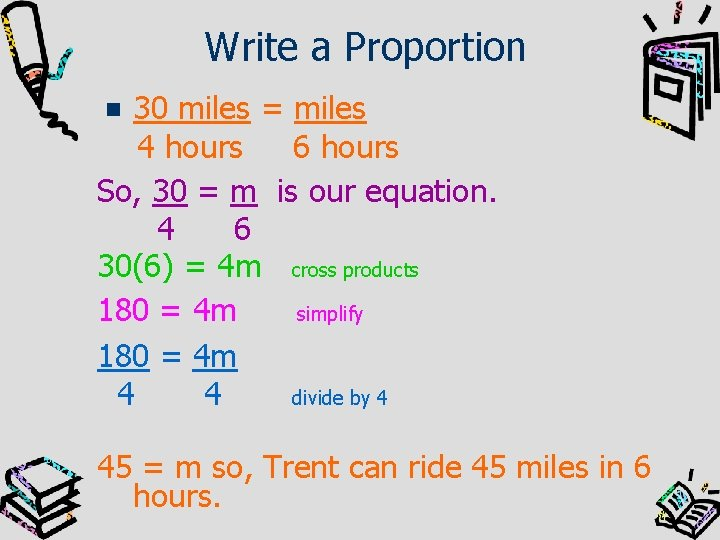Write a Proportion 30 miles = miles 4 hours 6 hours So, 30 = m is our equation. 4 6 30(6) = 4 m cross products 180 = 4 m simplify 180 = 4 m 4 4 divide by 4 45 = m so, Trent can ride 45 miles in 6 hours.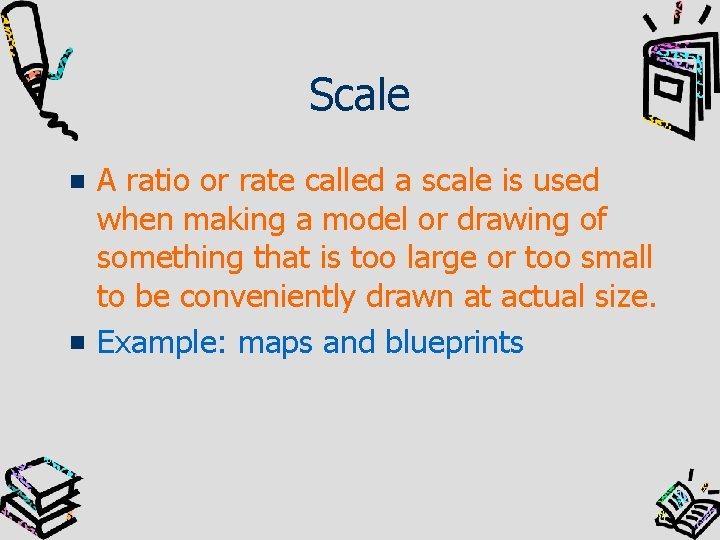Scale A ratio or rate called a scale is used when making a model or drawing of something that is too large or too small to be conveniently drawn at actual size. Example: maps and blueprintsUse a Scale Drawing In a road atlas, the scale for the map of Connecticut is 5 inches = 41 miles. What is the distance in miles represented by 2. 5 inches on the map? 5 = 2. 5 41 m 5 m = 2. 5(41) 5 m = 102. 5 5 5 m = 20. 5